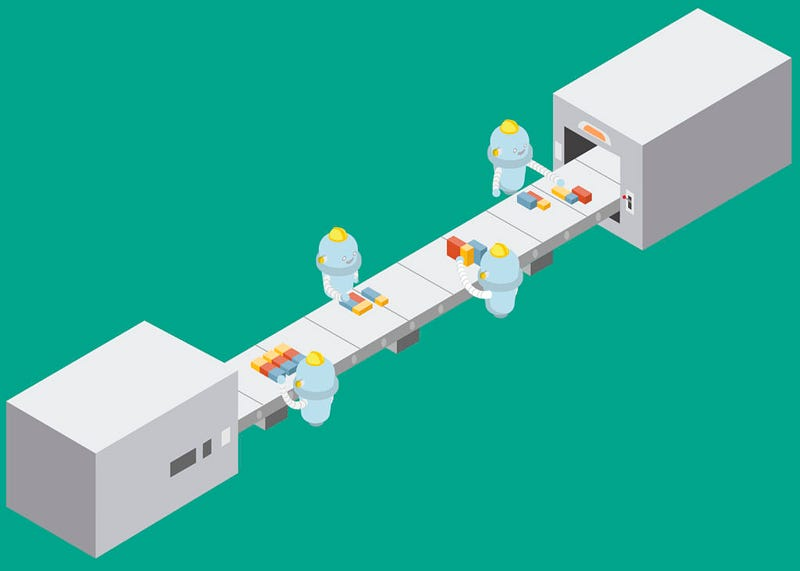# Machine Learning with Optimus on Apache Spark

The way most Machine Learning models work on Spark are not straightforward, and they need lots of feature engineering to work. That’s why we created the feature engineering section inside the Optimus Data Frame Transformer.commentsMachine Learning is one of the last steps, and the goal for most Data Science WorkFlows.

Some years ago the Apache Spark team created a library called MLlib where they coded great algorithms for Machine Learning.

Now with the ML library we can take advantage of the Dataframe API and its optimization to create easily Machine Learning Pipelines. Even though this task is not extremely hard, is not easy.

The way most Machine Learning models work on Spark are not straightforward, and they need lots of feature engineering to work.

That’s why we created the feature engineering section inside the Optimus Data Frame Transformer.

Installing Optimus:

To install optimus you just need to run:

`pip install optimuspyspark`

Importing OptimusML:

To import the Machine Learning Library you just need to import Optimus:

```# Importing Optimus
import optimus as op```

Remember you can combine the feature engineering methods we have with our Machine Learning Library.

Let’s take a look of what Optimus can do for you:

Logistic Regression for Text:

This method runs a logistic regression for input (text) DataFrame.

Let’s create a sample dataframe to see how it works.

```# Import Row from pyspark
from pyspark.sql import Row
# Importing Optimus
import optimus as op

df = op.sc. \
parallelize([Row(sentence='this is a test', label=0.),
Row(sentence='this is another test', label=1.)]). \
toDF()

df.show()```

```+-----+--------------------+
|label|            sentence|
+-----+--------------------+
|  0.0|      this is a test|
|  1.0|this is another test|
+-----+--------------------+```

And now let’s predict this labels with Optimus:

`df_predict, ml_model = op.ml.logistic_regression_text(df,"sentence")`

This instruction will return two things, first the DataFrame with predictions and also the other columns with steps used to build a pipeline and a Spark machine learning model where the third step (in the pipeline) will be the logistic regression.

The columns of df_predict are:

```df_predict.columns

['label',
'sentence',
'Tokenizer_4df79504b43d7aca6c0b__output',
'CountVectorizer_421c9454cfd127d9deff__output',
'LogisticRegression_406a8cef8029cfbbfeda__rawPrediction',
'LogisticRegression_406a8cef8029cfbbfeda__probability',
'LogisticRegression_406a8cef8029cfbbfeda__prediction']```

The names are long because those are the uid for each step in the pipeline. So lets see the prediction compared with the actual labels:

```transformer = op.DataFrameTransformer(df_predict)
transformer.select_idx([0,6]).show()```

```+-----+---------------------------------------------------+
|label|LogisticRegression_406a8cef8029cfbbfeda__prediction|
+-----+---------------------------------------------------+
|  0.0|                                                0.0|
|  1.0|                                                1.0|
+-----+---------------------------------------------------+```

So we just did ML with a single line in Optimus. Remember the model is exposed in the ml_model variable so you can save it and evaluate it.

N grams:

This method converts the input array of strings inside of a Spark DF into an array of n-grams. The default n is 2 so it will produce bi-grams.

Let’s create a sample dataframe to see how it works.

```# Import Row from pyspark
from pyspark.sql import Row,types
# Importing Optimus
import optimus as op

df = op.sc. \
parallelize([['this is the best sentence ever'],
['this is however the worst sentence available']])\

df_predict, model = op.ml.n_gram(df, input_col="sentence", n=2)```

The columns of df_predict are:

`df_predict.columns`

```['sentence',
'Tokenizer_4a0eb7921c3a33b0bec5__output',
'StopWordsRemover_4c5b9a5473e194516f3f__output',
'CountVectorizer_41638674bb4c4a8d454c__output',
'NGram_4e1d89fc70917c522134__output',
'CountVectorizer_4513a7ba6ce22e617be7__output',
'VectorAssembler_42719455dc1bde0c2a24__output',
'features']```

So lets see the bi-grams (we can change n as we want) for the sentences:

```transformer = op.DataFrameTransformer(df_predict)
transformer.select_idx().show()```

```+----------------------------------------------------+
|NGram_4e1d89fc70917c522134__output                  |
+----------------------------------------------------+
|[best sentence, sentence ever]                      |
|[however worst, worst sentence, sentence available] |
+----------------------------------------------------+```

And that’s it. N-grams with only one line of code.

Above we’ve been using the Pyspark Pipes definitions of Daniel Acuña, that he merged with Optimus, and because we use multiple pipelines we need those big names for the resulting columns, so we can know which uid correspond to each step.

Now let’s see other ML models you can build with OptimusML.